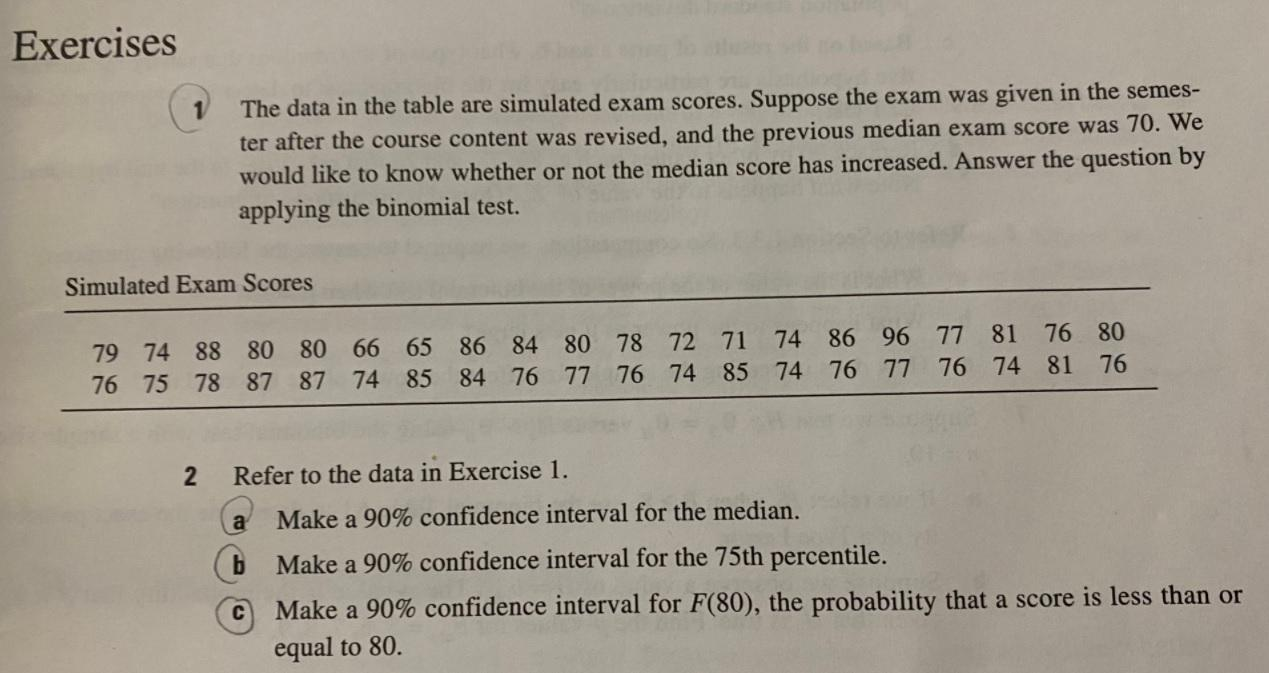# Question Solved1 Answerplease answer #1 and #2 using R studio and share codes. Exercises The data in the table are simulated exam scores. Suppose the exam was given in the semes- ter after the course content was revised, and the previous median exam score was 70. We would like to know whether or not the median score has increased. Answer the question by applying the binomial test. Simulated Exam Scores 79 74 88 80 80 66 65 86 84 80 78 72 71 74 86 96 77 81 76 80 76 75 78 87 87 74 85 84 76 77 76 74 85 74 76 77 76 74 81 76 2 Refer to the data in Exercise 1. a Make a 90% confidence interval for the median. Make a 90% confidence interval for the 75th percentile. Make a 90% confidence interval for F(80), the probability that a score is less than or equal to 80. сLJ6BYT The Asker · Probability and Statisticsplease answer #1 and #2 using R studio and share codes.

Transcribed Image Text: Exercises The data in the table are simulated exam scores. Suppose the exam was given in the semes- ter after the course content was revised, and the previous median exam score was 70. We would like to know whether or not the median score has increased. Answer the question by applying the binomial test. Simulated Exam Scores 79 74 88 80 80 66 65 86 84 80 78 72 71 74 86 96 77 81 76 80 76 75 78 87 87 74 85 84 76 77 76 74 85 74 76 77 76 74 81 76 2 Refer to the data in Exercise 1. a Make a 90% confidence interval for the median. Make a 90% confidence interval for the 75th percentile. Make a 90% confidence interval for F(80), the probability that a score is less than or equal to 80. с
More
Transcribed Image Text: Exercises The data in the table are simulated exam scores. Suppose the exam was given in the semes- ter after the course content was revised, and the previous median exam score was 70. We would like to know whether or not the median score has increased. Answer the question by applying the binomial test. Simulated Exam Scores 79 74 88 80 80 66 65 86 84 80 78 72 71 74 86 96 77 81 76 80 76 75 78 87 87 74 85 84 76 77 76 74 85 74 76 77 76 74 81 76 2 Refer to the data in Exercise 1. a Make a 90% confidence interval for the median. Make a 90% confidence interval for the 75th percentile. Make a 90% confidence interval for F(80), the probability that a score is less than or equal to 80. с